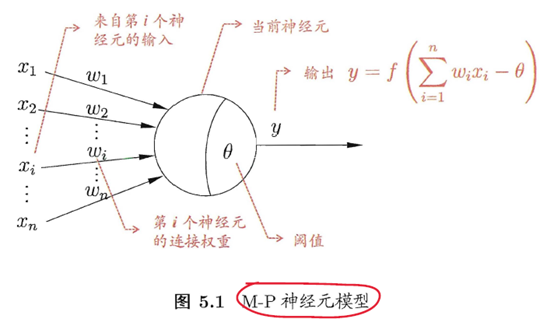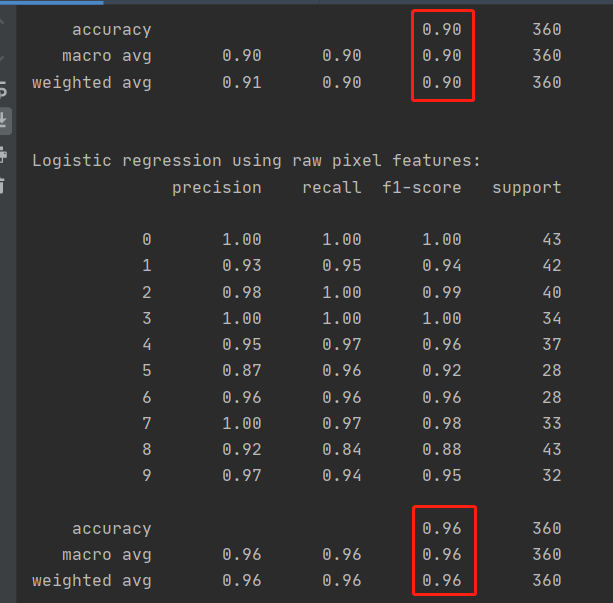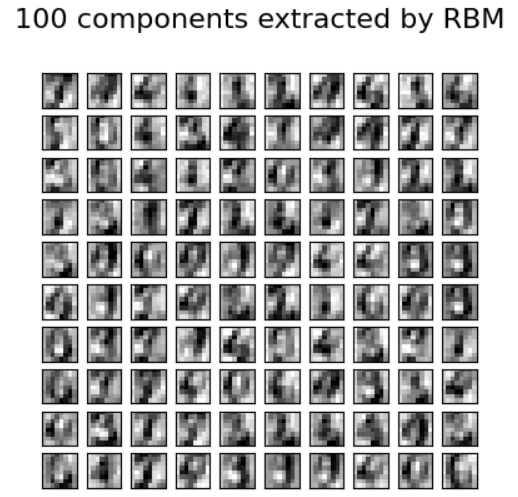# 机器学习之神经网络

## 1、术语

### 1.1、M-P 神经元模型### 1.3、误差逆传播算法

BP是一个迭代学习算法，可基于梯度下降(gradient descent)策略，以目标的负梯度方向对参数进行调整。
BP算法的工作流程

BP算法的目标是要最小化训练集D上的累积误差。

• 第一种策略是"早停" (early stopping): 将数据分成训练集和验证集，训练、集用来计算梯度、更新连接权和阀值，验证集用来估计误差，若训练集误差降低但验证集误差升高，则停止训练，同时返回具有最小验证集误差的连接权和阀值。
• 第二种策略是"正则化" (regularization) 基本思想是在误差目标函数中增加一个用于描述网络复杂度的部分，例如连接权与阀值的平方和。
跳出局部极小策略：使用随机梯度下降
与标准梯度下降法精确计算梯度不同，随机梯度下降法在计算梯度时加入了随机因素。即便陷入局部极小点，它计算出的梯度仍可能不为零，这样就有机会跳出局部极小继续搜索，找到全局最小。

### 1.4、其他常见神经网络

• RBF网络
RBF(Radial Basis Function，径向基函数)网络是一种单隐层前馈神经网络，使用径向基函数作为隐层神经元激活函数。
训练RBF 网络步骤：第一步，确定神经元中心，常用的方式包括随机采样、聚类等；第二步，利用BP算法等来确定参数。
• ART网络
竞争型学习(competitive learning) 是神经网络中一种常用的无监督学习策略，采用胜者通吃(winner-take-all)原则。ART(Adaptive Resonance Theory，自适应谐振理论)网络和SOM 网络是典型代表。
• SOM(Self-Organizing Map ，自组织映射)网络能将高维输入数据映射到低维空间，同时保持输入数据在高维空间的拓扑结构。
• 其他网络：级联相关网络，Elman 网络（递归），Boltzmann机（基于能量的递归）

### 1.5、深度学习

CNN 复合多套"卷积层"和"采样层"对输入信号进行加工，然后在连接层实现与输出目标之间的映射。每个卷积层都包含多个特征映射(feature map)，每个特征映射是一个由多神经元构成的平面，通过一种卷积滤波器提取输入的一种特征。采样层亦称为"汇合" (pooling)层，其作用是基于局部相关性原理进行亚采样，从而在减少数据量的同时保留有用信息。

## 2、Sklearn代码实现

Sklearn提供的是伯努利限制玻尔兹曼机(BernoulliRBM)，实现的训练算法被称为随机最大似然(Stochastic Maximum Likelihood ，SML)或持续对比发散(Persistent Contrastive Divergence ，PCD)。

import matplotlib.pyplot as plt
import numpy as np
from sklearn import datasets, linear_model, metrics
from sklearn.model_selection import train_test_split
from sklearn.neural_network import BernoulliRBM
from sklearn.pipeline import Pipeline
from sklearn.base import clone

if __name__ == '__main__':
# 加载手写字体数据集
digits = datasets.load_digits()
# 使用样本的所有特征
x = digits.data
y = digits.target
# 0-1 scaling
x = (x - np.min(x, 0)) / (np.max(x, 0) + 0.0001)
# 划分训练集和测试集
x_train, x_test, y_train, y_test = train_test_split(x, y, random_state=7, test_size=0.2)

# 创建模型
m_logistic = linear_model.LogisticRegression(solver='newton-cg', tol=1)
m_rbm = BernoulliRBM(random_state=0, verbose=True)
# 管道封装，步骤：BernoulliRBM，LogisticRegression
pipe_classifier = Pipeline(steps=[('rbm', m_rbm), ('logistic', m_logistic)])

# 训练
# 参数设置
m_rbm.learning_rate = 0.06
m_rbm.n_iter = 10
# More components tend to give better prediction performance, but larger fitting time
m_rbm.n_components = 100
m_logistic.C = 6000

# Training RBM-Logistic Pipeline
pipe_classifier.fit(x_train, y_train)
# Training the Logistic regression classifier directly on the pixel
raw_pixel_classifier = clone(m_logistic)
raw_pixel_classifier.C = 100.
raw_pixel_classifier.fit(x_train, y_train)

# 预测
y_pred = pipe_classifier.predict(x_test)
print("Logistic regression using RBM features:\n%s\n" % (metrics.classification_report(y_test, y_pred)))
y_pred = raw_pixel_classifier.predict(x_test)
print("Logistic regression using raw pixel features:\n%s\n" % (metrics.classification_report(y_test, y_pred)))

# Plotting
plt.figure(figsize=(4.2, 4))
for i, comp in enumerate(m_rbm.components_):
plt.subplot(10, 10, i + 1)
plt.imshow(comp.reshape((8, 8)), cmap=plt.cm.gray_r, interpolation='nearest')
plt.xticks(())
plt.yticks(())
plt.suptitle('100 components extracted by RBM', fontsize=16)
plt.subplots_adjust(0.08, 0.02, 0.92, 0.85, 0.08, 0.23)

plt.show()09-011万+
03-17974505-12291
05-1848
11-031470
04-05115
03-271938
10-231万+
06-085万+
05-246744
05-302946
08-113万+
12-222万+
05-124786
11-232万+
08-08298
05-021039
12-011万+
12-1213万+
04-102万+
©️2020 CSDN 皮肤主题: 大白 设计师:CSDN官方博客